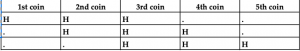## Example 1:

### Solution:

Suppose you flip a coin. What is the chance Heads will appear?50% (i.e. 1/2)
What is the chance that tails will appear? 50% (i.e. 1/2)

## Example 2:

Toss a dice. What is the probability that an even number will appear?

### Solution:

The six numbers on a dice are 1, 2, 3, 4, 5, 6.
On the dice, 2, 4 and 6 are the even numbers.
So, the probability an even number will fall is 3/6 i.e. ½

## Example 3:

In a bag, there are 3 green and 4 red marbles. Take out any two marbles out of the bag. What is the probability the two marbles are green-colored?

### Solution:

Bag contains 7 marbles. 2 marbles are taken out. 2 out of 7 can be taken out in 7c2 ways i.e. 21 ways. (Recall that r things can be selected out of n things in ncr ways from combinations). 2 green out of 3 green marbles can be taken out in 3c2 ways i.e. 3 ways. The probability is therefore: 3/21 = 1/7.

From the three examples above, we can define probability as follows: If E denotes an event, then the probability Event E will happen is: P (E) = (Outcomes favorable for E)/ (Total number of outcomes). The table below summaries the salient points in the 3 examples above.

So, try a definition for Probability? Here we go:
Let E denote an Event and P (E) the probability that Event E will happen. Then, P (E) = (Number of outcomes favorably for E)/(Total number of outcomes)

Solved Examples:
Five coins are tossed. Find the probability of: 3 heads; At least 3 heads; At most 2 heads; 3 heads, exactly next to each other.

### Solution:

Use the above formula: P (E) = (Number of outcomes favorable for E)/(Total number of outcomes)
First find the total number of outcomes when 5 coins are tossed.
It is 2 × 2 × 2 × 2 × 2 × 2 = 25 = 32 outcomes and not 2 × 5 = 10 outcomes.
(If 3 coins are tossed, the total number of outcomes is 2 × 2 × 2 = 8 outcomes and not
2 × 3 = 6 outcomes.)How, as follows:

So, 3 coins give 2 × 2 × 2 = 23 = 8 outcomes.
Likewise, 5 coins give 2 × 2 × 2 × 2 × 2 = 25 = 32 outcomes.
Now, how many outcomes show 3 heads out of 32?
5c3 i.e. 10 outcomes. How? As follows:

The dots indicate Tails. Tabulating is difficult. You must use an easier way to find favorable outcomes, with a formula probably.
And it is combinations formula: ncr

We need 3 Heads. Which 3 coins must show the required 3 Heads?
Any 3 coins. So, select any 3 coins out of the 5 coins. In how many ways?
Yes, in ncr ways, i.e. 5c3 ways i.e. 10 ways. Each way is one outcome, 10 ways are 10 outcomes.
So, the probability 3 Heads will appear is = 10/32 = 5/16

So, P (At least 3 Heads) = P (3H) or P (4H) or P (5H) (Remember one rule: Write + for Or when it needs to used and × for And)
So, P (At least 3 Heads) = P (3H) + P (4H) + P (5H) (0.5 and 0.5)

Probability of at most 2 Heads:
At most means maximum 2 heads i.e. up to 2 heads, i.e. less than or equal to 2 heads.